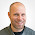19 Aug 2013

Text Mining with R - Comparing Word Counts in Two Text Documents

Here's what I came up with to compare word counts in two pieces of text. If you got any idea, I'd love to learn about alternatives!

```## a function that compares word counts in two texts
wordcount <- function(x, y, stem = F, minlen = 1, marg = F) {

require(tm)

x_clean <- unlist(strsplit(removePunctuation(x), "\\s+"))
y_clean <- unlist(strsplit(removePunctuation(y), "\\s+"))

x_clean <- tolower(x_clean[nchar(x_clean) >= minlen])
y_clean <- tolower(y_clean[nchar(y_clean) >= minlen])

if ( stem == T ) {

x_stem <- stemDocument(x_clean)
y_stem <- stemDocument(y_clean)
x_tab <- table(x_stem)
y_tab <- table(y_stem)

cnam <- sort(unique(c(names(x_tab), names(y_tab))))

z <- matrix(rep(0, 3*(length(cnam)+1)), 3, length(cnam)+1, dimnames=list(c("x", "y", "rowsum"), c(cnam, "colsum")))
z["x", names(x_tab)] <- x_tab
z["y", names(y_tab)] <- y_tab
z["rowsum",] <- colSums(z)
z[,"colsum"] <- rowSums(z)
ifelse(marg == T, return(t(z)), return(t(z[1:dim(z)-1, 1:dim(z)-1])))

} else {

x_tab <- table(x_clean)
y_tab <- table(y_clean)

cnam <- sort(unique(c(names(x_tab), names(y_tab))))

z <- matrix(rep(0, 3*(length(cnam)+1)), 3, length(cnam)+1, dimnames=list(c("x", "y", "rowsum"), c(cnam, "colsum")))
z["x", names(x_tab)] <- x_tab
z["y", names(y_tab)] <- y_tab
z["rowsum",] <- colSums(z)
z[,"colsum"] <- rowSums(z)
ifelse(marg == T, return(t(z)), return(t(z[1:dim(z)-1, 1:dim(z)-1])))
}
}

## example
x = "Hello new, new world, this is one of my nice text documents - I wrote it today"
y = "Good bye old, old world, this is a nicely and well written text document"

wordcount(x, y, stem = T, minlen = 3, marg = T)
```

Follow-Up:

Thanks a lot for the comments! As I'm not that much into text mining I was trying to reinvent the wheel (in a rather dilettante manner) - missing the capabilities of existing packages. Here's the shortest code that I was able to find doing the same thing (with the potential to get out much more of it, if desired).
```x = "Hello new, new world, this is one of my nice text documents"
y = "Good bye old, old world, this is a text document"
z = "Good bye old, old world, this is a text document with WORDS for STEMMING  - BTW, what is the stem of irregular verbs like write, wrote, written?"

# make a corpus with two or more documents (the cool thing here is that it could be endless (almost) numbers
# of documents to be cross tabulated with the used terms. And the control function enables you
# to do lots of tricks with it before it will be tabulated, see ?termFreq, i.e.)

xyz <- as.list(c(x,y,z))
xyz_corp <- Corpus(VectorSource(xyz))

cntr <- list(removePunctuation = T, stemming = T, wordLengths = c(3, Inf))

as.matrix(TermDocumentMatrix(xyz_corp, control = cntr))
```

1.What you're doing is a term document matrix (the tm package) or what the qdap
package calls a word frequency matrix. Here is a demo of this functionality in the qdap package: https://gist.github.com/trinker/6275776

1.Very helpful - thanks a lot!

2.Hi
Nice function! If you have more stuff like that to do, you may be interested in this book: . (Yes, I'm self-advertising, but I see so few people out there using R for text processing that I am always happy to see someone do this with R and not with Perl/Python that I can't resist.)

1.I'm afraid the link got lost somewhere..

2.I would actually be interested to hear about any book related to R and Text Mining /NLP topics.

3.Hi dude, saw your post on R bloggers, why dont you try using frequency function built into tm package. I think it is findFreq. I guess it will save a lot of line of codes and be more efficient for larger datasets. Let me know if I have missed something. I blog about R & using Twitter as a data source, would love to here your thoughts and suggestions. http://tweetsent.wordpress.com/

1.I will surely take a look at this function - however, I wonder if it will be able to deal with several documents and to combine the result in one df?